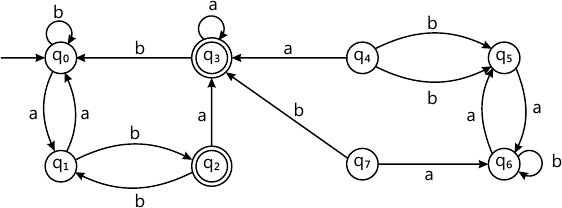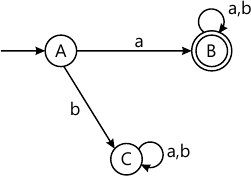MORE IN Theory of Computation
SPPU Information Technology (Semester 5)
Theory of Computation
December 2014
Total marks: --
Total time: --
INSTRUCTIONS
(1) Assume appropriate data and state your reasons
(2) Marks are given to the right of every question
(3) Draw neat diagrams wherever necessary

Answer any one question from Q1 and Q2
1 (a) Write the formal definition of the following:
i) Finite Automata
ii) E-closure
4 M
1 (b) Using pumping lemma for the regular sets prove the language [ L={ a^{i^2} | i ge | } ] is not regular.
6 M

2 (a) Construct the minimum state automation equivalent to the transition diagram given as below:6 M
2 (b) Construct Regular Expression for the following transition diagram using Arden's theorem.4 M

Answer any one question from Q3 and Q4
3 (a) Construct the parse trees for the strings using specified derivation for the given grammar G. [ G=({ S,A,B}, {a,b}, P, {S}) \ egin {align*} P= {&S o aB, S o bA} \ & A o a, A o aS, A o bAA \ &B o b, B o bS, B o aBB end{align*} ] Strings: i) aaabbb (rightmost derivation)
ii) aababb (rightmost derivation).
4 M
3 (b) Describe CFG, Chomsky Normal Form and Greibach Normal Form, with suitable examples.
6 M

4 (a) Remove Unit-productions from the given grammar.
P = { S → ABA|BA|AA|AB|A|B
A → aA|a
B → bB|b
}
4 M
4 (b) Define ambiguous grammar.
1) S ? 0S|S|1S0S| ∈
2) S → AA
A → aAb|bAa| ∈
Consider above grammars. Test whether these grammars are ambiguous.
6 M

Answer any one question from Q5 and Q6
5 (a) Construct a PDA that accepts the following language using CNF. [ L={a^{2n} | n ge 0 }. ]
8 M
5 (b) Write formal definition of PDA. Explain its elements. What are different types of PDA? What are the applications of PDA?
8 M

6 (a) Design a PDA accepting {anbman|m,n≥1} simulate a PDA for the string 'aabaa'.
8 M
6 (b) Define post machine. Explain its elements. Show that the post machine is more powerful that PDA.
8 M

Answer any one question from Q7 and Q8
7 (a) Design a TM which accepts all strings of the form anbn for n≥1 and rejects all other strings. Draw the transition diagram. Simulated TM for some string.
10 M
Write short notes on:
7 (b) (i) Universal Turing Machine.
4 M
7 (b) (ii) Multi-tape Turing Machine.
4 M

8 (a) Design a TM to add two unary numbers.
8 M
8 (b) Design a TM that recognize a string containing aba as a substring.
6 M
8 (c) Write a short note on Non deterministic Turing Machine.
4 M

Answer any one question from Q9 and Q10
9 (a) Show that for two recursive languages L1 &L2, each of the following is recursive
i) L1 ∪ L2
ii) L1 ∩ L2
8 M
9(b) Define decidability. How to prove the given language is undecidable problems.
8 M

10 (a) Write a short note on halting problem of Turing Machine.
8 M
Explain the following
10 (b)(i) Recursive sets.
4 M
10 (b)(ii) Turing reducibility.
4 M

More question papers from Theory of Computation